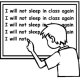Home > Grade 8 > Square Root Expression

# Square Root Expression

Directions: Using the digits 1 to 9, at most one time each, fill in the boxes to make the following expression as close to 0 as possible.### Hint

What are all the perfect square three digit numbers? Several of these can’t be used since they contain a repeated digit (e.g. 121 uses the digit 1 twice and 144 uses the digit 4 twice)

Using Perfect squares:
Sqrt(256) – sqrt(81) – sqrt(9) = 4

Using non-perfect squares:
Sqrt(145) – sqrt(92) – sqrt(6) = 0.00044178938368

Source: Erick Lee

## Equations of Perpendicular Lines

Directions: Using the integers -9 to 9 (excluding 0) at most one time each, fill …

1.Dang it.

I got:
√124 – √89 – √3 = -0.030503214

•You tried. That is what is important!

•Good job!! It doesn’t matter if you make a mistake! The important thing is that you tried! You did your personal best and that is what matters!!! Just keep trying and you will make a good answer eventually!! Just do your personal best and if you get it wrong than don’t worry about it! it is fine! Keep on trying!

2.Should it not be √256 – √81 -√49 = 0 ?

•Your answer looks correct. Maybe the wording needs to be clarified? It says as close to zero, maybe the question needs to state without equaling zero?

•The last term has space for only one digit, so √49 doesn’t work.

•I thought there could only be one digit square root on the last term?

•Correct Kathy and Suzanne. Unfortunately Kristen, the last root must have a single digit.

3.4.√121-√81-√4
The answer to this equation would be 0

•You can only use the digit “1” at most once.

5.or 11-9-2 when It is simplified

6.or √121-√64-√9
The answer would be 0 as well

7.you are repeating the number 1 , 121 has two and then theres a third “1” in 81

8./121-/64-/9=0

9.Abbey Turrentine

257-81-49=4

10.11.|121|-|81|-|4|

12.|121|-|81|-|5|

13.|121|-|82|-|5|

14.Spencer Turrentine

121-81-9= 4

15.I was working on this problem in preparation for having my 8th grade students work on it. I assumed that I should place digits so that the digits under each radical formed a perfect square, but then I realized the directions didn’t explicitly state that. I am interested in hearing how teachers have used this problem in their classroom and the discussions that resulted.